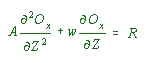### OCEANIC VERTICAL REDOX CALCULATORafter Wilde (1987)

CLIMATE:ATMOSPHERE
Glacial
Pre-Post Glacial
Non Glacial
PAL%mL/L

#### Average Redox through the PycnoclineAverage Redox over 5000 meters

Ts Depth DO mL/L
a
R0
A C1
C2
C3
Weighted String

D.O. at Specific Depth within the Pycnocline
DepthmetersDOmL/L

The model assumes that the oxidative decay proceeds (Riley, 1951; Wyrtki, 1962, p. 13) as:

R = Roe-aZ.............................. (1)
Where
R = rate of decay at depth Z
Ro = rate at the surface
a = function of oxidized substance
Z = depth

and the steady state concentration of oxygen (Ox) can be found by the solution of:......................................(2)
Where
A = vertical exchange coefficient

Wyrtki (1961, p. 44) has shown how the temperature profile can be used to calculate w/A from the expression:

wT - A T/z = wTD................... (3)

Where
T = potential temperature at given depth
TD= temperature at the depth of ascending water (base of the thermocline)

assuming, within the main thermocline, that (1) w is constant and (2) A is at a minimum. For the central part of the modern ocean he estimated that w = 2 x10-5 cm/sec and A = 0.5 cm2/sec were reasonable.

As noted in the text in eq (2) the concentration of oxygen can be modeled as:......................................(2)
Where
A = vertical exchange coefficient
R = RoeaZ

The solution of eq (2) for Os (oxidant) is:

Ox=C1 + C2e-(w/A)Z + C3e-aZ

where the constants of integration are:

C1 = Ox (@ upper boundary) - C2e-(w/A)Z (@upper boundary) - C3e-aZ(@ upper boundary);

C2 = [{Ox (@ upper boundary)--Ox (@ lower boundary)} -
{C3e-aZ (@ Upper boundary) +C3e-aZ (@ Lower boundary)} /
{e-(w/A)Z (@ Upper boundary) - e-(w/A)Z (@ Lower boundary)} ];

C3 = Ro/Aa(a - w/A)

Taken as constants are a = 0.000025 and Ro = 7.5 X 10-9.

Taken as constant for a particular climate (table 1) is the ratio (w/A), which is related to variation in temperature in the thermocline.